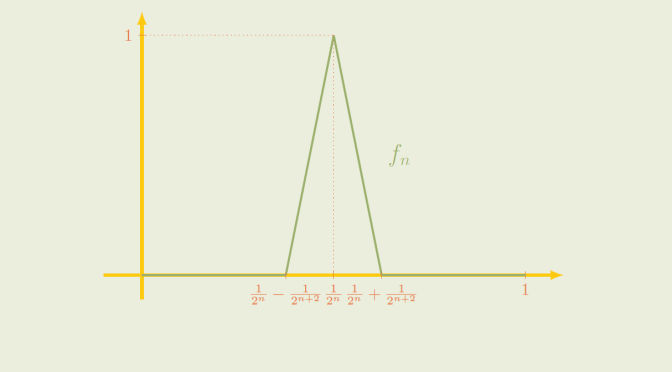# A non-compact closed ball

Consider a normed vector space $$(X, \Vert \cdot \Vert)$$. If $$X$$ is finite-dimensional, then a subset $$Y \subset X$$ is compact if and only if it is closed and bounded. In particular a closed ball $$B_r[a] = \{x \in X \, ; \, \Vert x – a \Vert \le r\}$$ is always compact if $$X$$ is finite-dimensional.

### The space $$A=C([0,1],\mathbb R)$$

Consider the space $$A=C([0,1],\mathbb R)$$ of the real continuous functions defined on the interval $$[0,1]$$ endowed with the sup norm:
$\Vert f \Vert = \sup\limits_{x \in [0,1]} \vert f(x) \vert$
Is the closed unit ball $$B_1$$ compact? The answer is negative and we provide two proofs.

The first one is based on open covers. For $$n \ge 1$$, we denote by $$f_n$$ the piecewise linear map defined by $\begin{cases} f_n(0)=f_n(\frac{1}{2^n}-\frac{1}{2^{n+2}})=0 \\ f_n(\frac{1}{2^n})=1 \\ f_n(\frac{1}{2^n}+\frac{1}{2^{n+2}})=f_n(1)=0 \end{cases}$ All the $$f_n$$ belong to $$B_1$$. Moreover for $$1 \le n < m$$ we have $$\frac{1}{2^n}+\frac{1}{2^{n+2}} < \frac{1}{2^m}-\frac{1}{2^{m+2}}$$. Hence the supports of the $$f_n$$ are disjoint and $$\Vert f_n – f_m \Vert = 1$$.

Now consider the open cover $$\mathcal U=\{B_{\frac{1}{2}}(x) \, ; \, x \in B_1\}$$. For $$x \in B_1\}$$ and $$u,v \in B_{\frac{1}{2}}(x)$$, $$\Vert u -v \Vert < 1$$. Therefore, each $$B_{\frac{1}{2}}(x)$$ contains at most one $$f_n$$ and a finite subcover of $$\mathcal U$$ will contain only a finite number of $$f_n$$ proving that $$A$$ is not compact.

Second proof based on convergent subsequence. As $$A$$ is a metric space, it is enough to prove that $$A$$ is not sequentially compact. Consider the sequence of functions $$g_n : x \mapsto x^n$$. The sequence is bounded as for all $$n \in \mathbb N$$, $$\Vert g_n \Vert = 1$$. If $$(g_n)$$ would have a convergent subsequence, the subsequence would converge pointwise to the function equal to $$0$$ on $$[0,1)$$ and to $$1$$ at $$1$$. As this function is not continuous, $$(g_n)$$ cannot have a subsequence converging to a map $$g \in A$$.

### Riesz’s theorem

The non-compactness of $$A=C([0,1],\mathbb R)$$ is not so strange. Based on Riesz’s lemma one can show that the unit ball of an infinite-dimensional normed space $$X$$ is never compact. This is sometimes known as the Riesz’s theorem.

The non-compactness of $$A=C([0,1],\mathbb R)$$ is just standard for infinite-dimensional normed vector spaces!